# It would be very useful to have a theory about computability of functions R" -> R. Given that there Q.2 are an u...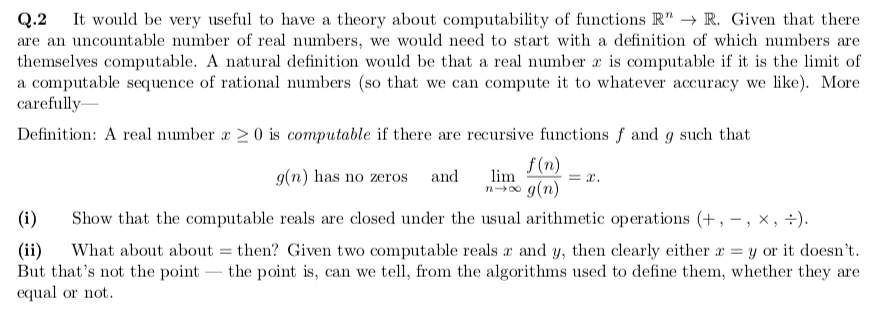It would be very useful to have a theory about computability of functions R" -> R. Given that there Q.2 are an uncountable number of real numbers, we would need to start with a definition of which numbers are themselves computable. A natural definition would be that a real number x is computable if it is the limit of computable sequence of rational numbers (so that we can compute it to whatever accuracy we like). More carefully V a Definition: A real number x 2 0 is computable if there are recursive functions f and g such that f (n) g(n) has no zeros lim and = g(n) Show that the computable reals are closed under the usual arithmetic operations , -, x, -) (i) y or it doesn't (ii) But that's not the point - the point is, can we tell, from the algorithms used to define them, whether they equal or not What about about then? Given two computable reals and y, then clearly either are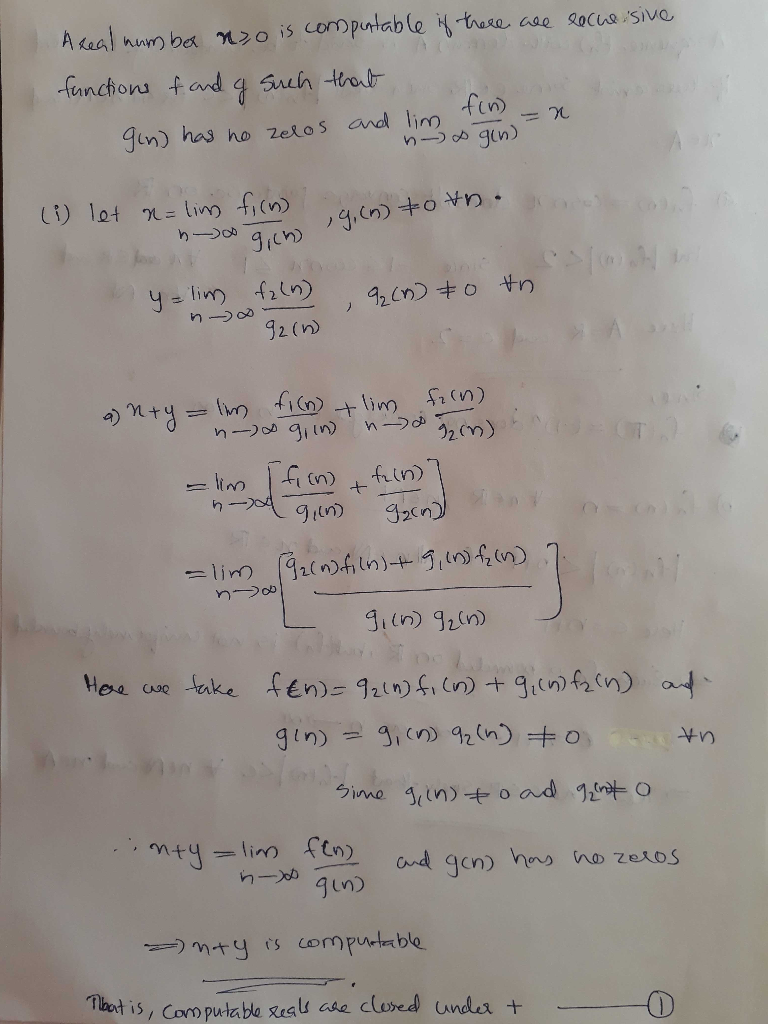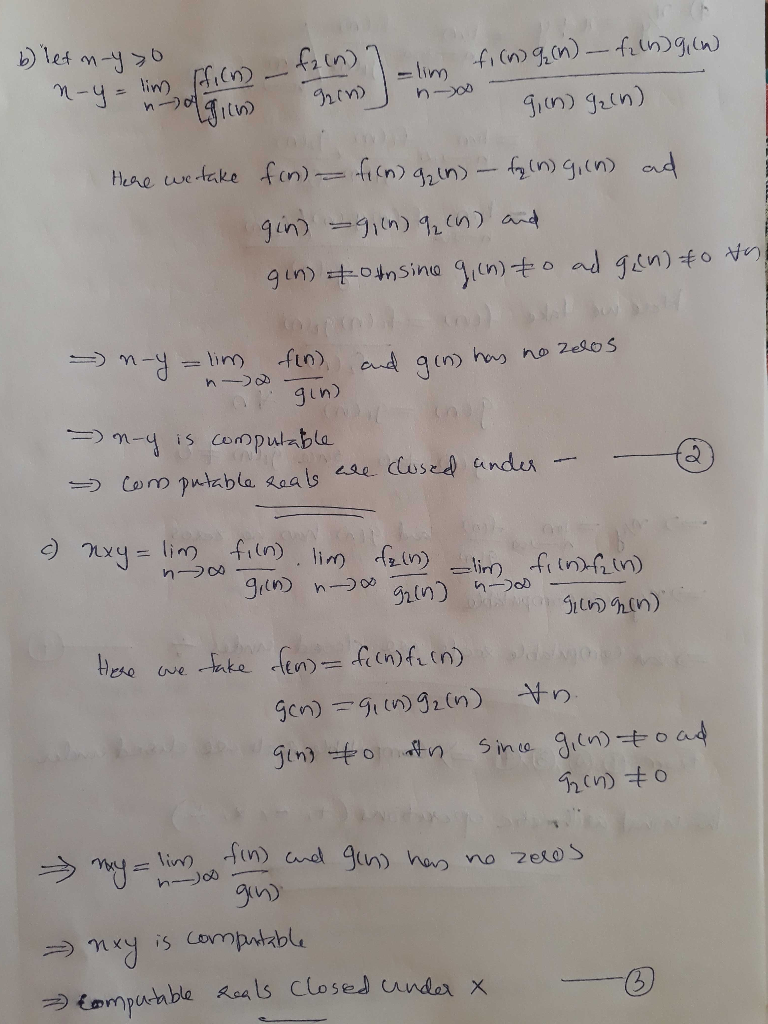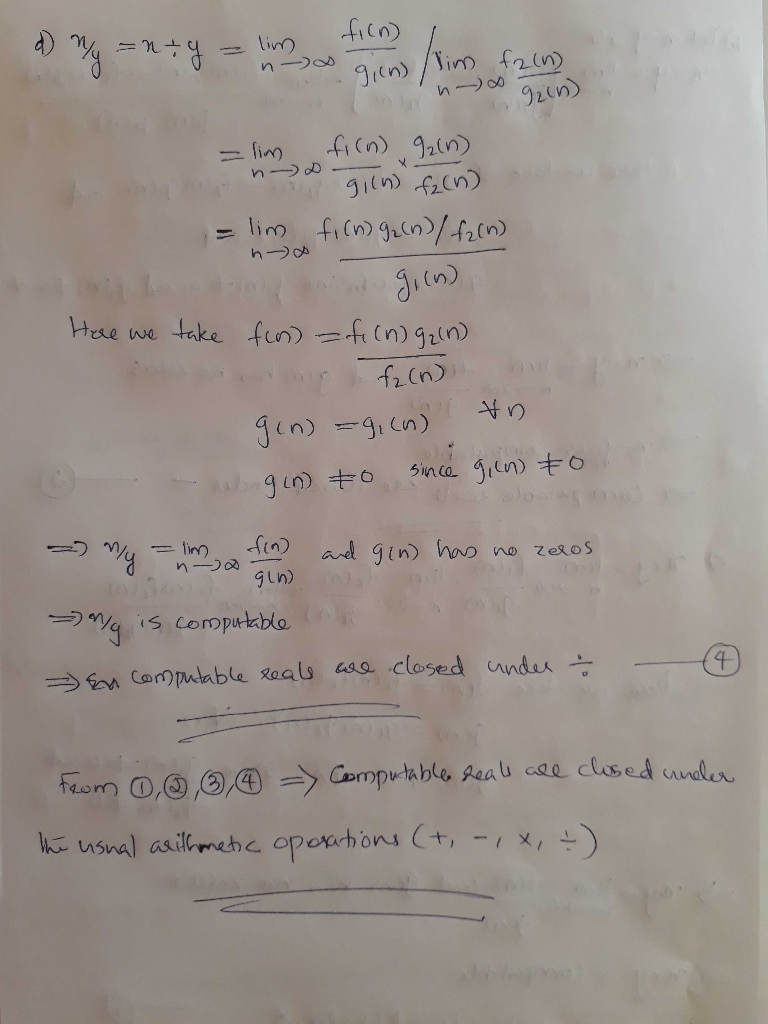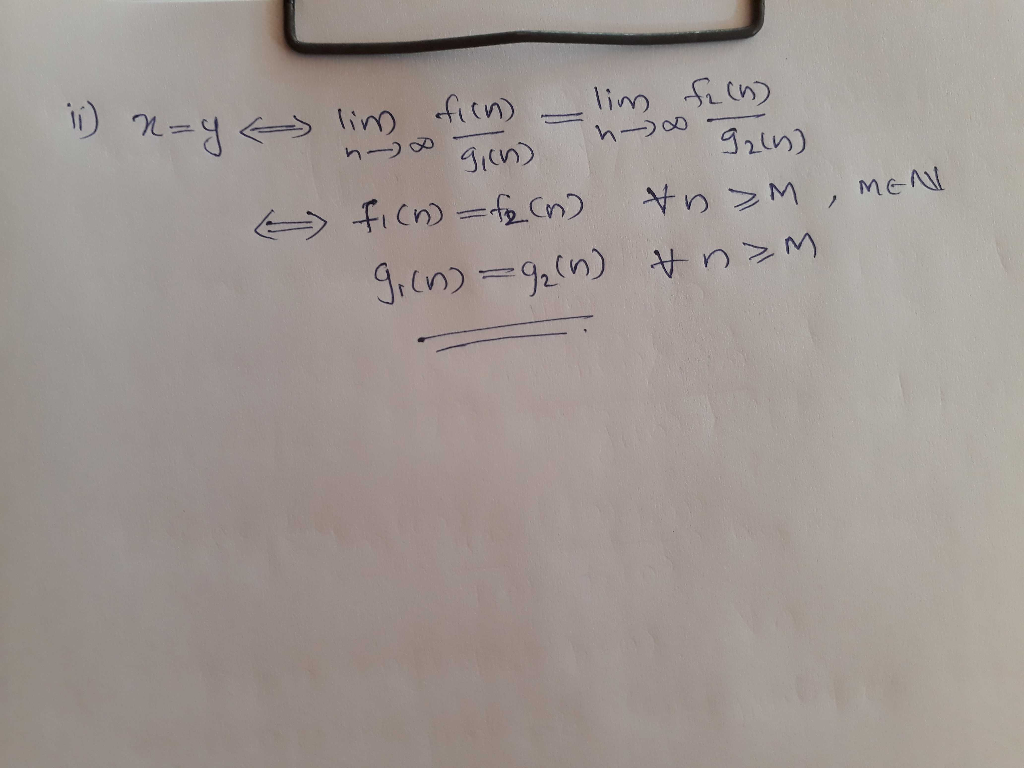##### Add Answer of: It would be very useful to have a theory about computability of functions R" -> R. Given that there Q.2 are an u...
More Homework Help Questions Additional questions in this topic.